•
•
•
•
•
•
•
•

# GALILEAN AND LORENTZ TRANSFORMATION

Today we are going to talk about a very important concept that is the Galilean and Lorentz Transformation and this concept of transformation is very important for the mathematical explanation of relativistic physics. When Einstein put his special theory of relativity in September 1905. Then various new concepts get born out of this theory, that is the time dilatation, length contraction, mass-energy equivalence, relativistic mass. And all these new concepts can only be explained by using mathematics.

So today we are going to take a look at this foundation mathematics of special theory of relativity, without which special theory of relativity is somehow incomplete. So Let’s starts our talk with Galilean transformation.

## GALILEAN TRANSFORMATIONConsider the two frames of reference S and S’ having the coordinates of any point P is (x,y,z) and (x’, y’,z’), initially both frames of reference are at the same position i.e. coincide with each other, where t is zero for both references when they are at the initial stage.

Then if the second frame S’ starts moving in the direction of the x-axis with some constant velocity v with respect to the first frame of reference S. (The first frame is a fixed frame of reference).

Here you have to remember that this velocity is very very very small in comparison to the speed of light. This is a day-to-day life velocity. Galilean Transformation is applicable only for small moving frame velocities.

If the second frame is moving away from the fixed frame S with v velocity then the time elapsed for any observer which is present at fixed frame S will be “t” but for the observer which is in the moving frame will be t’.

And we know from the above data that the distance of point P from first frame S is x, and from the second frame S’ is x’. Then the distance traveled by the second frame S’ w.r.t first frame S is given by the product of velocity v and time t ie. vt. (Here v and t both respect to S).
Then the distance between the point P and second frame S’ is given as the difference of total distance from S ie. x and traveled distance i.e. vt. x’ = x – vt.   if second frame S’ starts moving towards the fixed frame S, then total distance x is given as-
x = x’ + vt’
And if S’ is moving along the x-axis only then y and z coordinates of point P are not changing, the only x undergoes changes because the frame is moving along the x-axis.
It means y’ = y and z’ =z, and if velocity is very small then t and t’ will also same and there will be no difference in time.
Then the elements of fixed frame S are related to the elements of moving frame S’ are as follows.
x’ = x – vt, y’ = y, z’ = z, and t’ = t
This relationship is called Transformation, and this Transformation is given by Galileo so it is called Galilean transformation. Now let’s talk about Lorentz Transformation.

## LORENTZ TRANSFORMATION

We have talked about the Galilean transformation above, which is applicable only for small velocity. In which S’ is moving with small velocity v wrt fixed frame S. But what would happen if second frame S’ starts moving with the approx of the speed of light.

If it is moving with the speed of light then the difference between the t for the fixed frame and t’ for moving frame is significant because time becomes very very slow for moving frame w.r.t to fixed rest frame, which is called time dilation. Now let’s talk about the Lorentz Transformation.

### DERIVATION FOR LORENTZ TRANSFORMATIONS

Consider the two frames of reference S and S’ having the coordinates of any point P is (x,y,z,t) and (x’, y’,z’,t’), initially both frames of reference are at the same position ie. coincide to each other, where t is zero for both references when they are at the initial stage.

Then if the second frame S’ starts moving in the direction of the x-axis with some constant velocity v with respect to the first frame of reference S. And this velocity v is approximately equal to the speed of light, (the first frame is a fixed frame of reference).

Now think that from a light source, a beam of light is to be focused on the point P from the origin of the fixed frame of reference S. And the same light beam is also focused on the point P from the origin of the moving frame of reference S’.

And we know from the above data that the distance of point P from first frame S is x, and from the second frame S’ is x’.Then these distances can also be expressed as: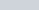, from the first frame of reference S, and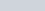, from the second frame of reference S’, which is moving.andfrom the galilean transformation we know that,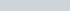, and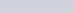But in Lorentz Transformation this relation is written as: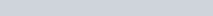Where k is some constant.
Multiplying these two  equations we get,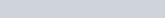Putting the value of x and x’ in the multiplication, we get,And finally we get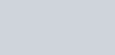and this value of constant k is called Lorentz factor which is denoted by γ.
And  So,This is a very important result because it is very helpful to derive the expression for time dilation, relativistic mass, length contraction. So this is all about Galilean and Lorentz transformation.